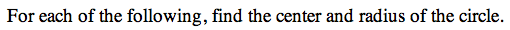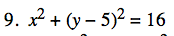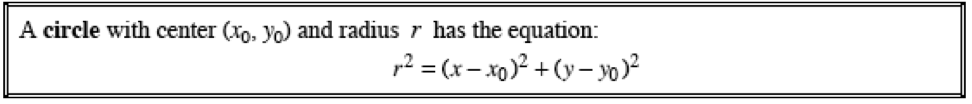13C-9.

### Missing problem for Book PCT => Chapter Ch13 => Lesson 13.1.1 => Problem 13C-9

Created from orphaned homework help problemIf r² = 16, what is r?
How is the center affected by the equation?